# Year 2020

The four-digit number divided by 2020 gives a result of 1, **. (Can not be in form 1,*0. ) Write all the options.

Result

n1 =  2323
n2 =  2525
n3 =  2727
n4 =  2929
n5 =  3131
n6 =  3333
n7 =  3535
n8 =  3737
n9 =  3939

#### Solution:

$n_{ 1 } = 2323 \ \\ d_{ 1 } = n_{ 1 }/2020 = 2323/2020 = \dfrac{ 23 }{ 20 } = 1.15$
$n_{ 2 } = 2525 \ \\ d_{ 2 } = n_{ 2 }/2020 = 2525/2020 = \dfrac{ 5 }{ 4 } = 1.25$
$n_{ 3 } = 2727 \ \\ d_{ 3 } = n_{ 3 }/2020 = 2727/2020 = \dfrac{ 27 }{ 20 } = 1.35$
$n_{ 4 } = 2929 \ \\ d_{ 4 } = n_{ 4 }/2020 = 2929/2020 = \dfrac{ 29 }{ 20 } = 1.45$
$n_{ 5 } = 3131 \ \\ d_{ 5 } = n_{ 5 }/2020 = 3131/2020 = \dfrac{ 31 }{ 20 } = 1.55$
$n_{ 6 } = 3333 \ \\ d_{ 6 } = n_{ 6 }/2020 = 3333/2020 = \dfrac{ 33 }{ 20 } = 1.65$
$n_{ 7 } = 3535 \ \\ d_{ 7 } = n_{ 7 }/2020 = 3535/2020 = \dfrac{ 7 }{ 4 } = 1.75$
$n_{ 8 } = 3737 \ \\ d_{ 8 } = n_{ 8 }/2020 = 3737/2020 = \dfrac{ 37 }{ 20 } = 1.85$
$n_{ 9 } = 3939 \ \\ d_{ 9 } = n_{ 9 }/2020 = 3939/2020 = \dfrac{ 39 }{ 20 } = 1.95$

Leave us a comment of this math problem and its solution (i.e. if it is still somewhat unclear...):Be the first to comment!#### Following knowledge from mathematics are needed to solve this word math problem:

See also our variations calculator. Would you like to compute count of combinations?

## Next similar math problems:

1. Seven-segmet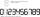Lenka is amused that he punched a calculator (seven-segment display) numbers and used only digits 2 to 9. Some numbers have the property that their image in the axial or central symmetry was again give some number. Determine the maximum number of three-d
2. Pages countingThere are pages numbered from 2 to 104 in the book. How many digits have to be printed to number the pages?
3. RatioIncrease in the ratio 20:4 number 18.5.
4. Find the 11Find the quotient of 229.12 and 12.32
5. Divide 5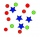Divide 288 in the following ratio 3 : 4 : 5
6. Fewer than 500 sheep,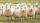There are fewer than 500 sheep, but if they stand in a double, triple, quadruple, five and sixth order, one sheep will remain. But they can stand in the seventh order. How many are sheep?
7. To improve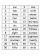To improve her handwriting Paula practices writing the numbers 1 to 200 in words. How many times will she have written the word "one" in all?
8. Homework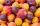In the crate are 18 plums, 27 apricot and 36 nuts. How many pieces of fruit left in the crate when Peter took 8 ninth: 1. nuts 2. apricots 3. fruit 4. drupe
9. Arble bagA marble bag sold by Rachel's Marble Company contains 5 orange marbles for every 6 green marbles. If a bag has 35 orange marbles, how many green marbles does it contain?
10. Strange x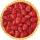For what x is true ??
11. Percent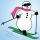From 99 children who participated in the ski course were 23 excellent skiers, 13 good and 20 average and the rest were beginners. Calculate this data in percentages.
12. Log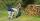Worker cut his thick log to 6 pieces for 30 min. How long he cut log to 12 pieces?
13. Shirt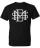Mrs. Vítková bought each of his three children the same shirt paid CZK 1,000. Saleswoman she returned 568,60 CZK. What was the price of one shirt?
14. Seven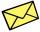Seven friends agree to send everyone a holiday card. How many postcards were sent?
15. FactoryIn the factory workers work in three shifts. In the first inning operates half of all employees in the second inning and a third in the third inning 200 employees. How many employees work at the factory?
16. Percents - easyHow many percent is 432 out of 434?
17. ClassIn a class are 32 pupils. Of these are 8 boys. What percentage of girls are in the class?